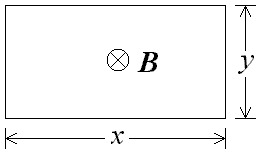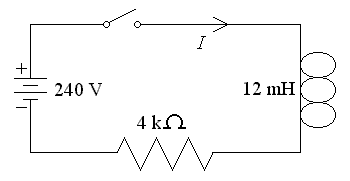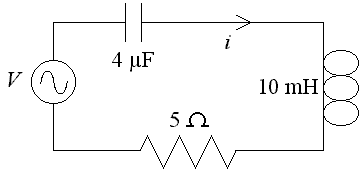First Name: ___________________ Last Name: ____________________ Section: _________

March 24, 2000 Physics 208

Exam 2

Print your name and section clearly on all five pages. (If you do not know your section number, write your TAs name.) Show all work in the space immediately below each problem. Your final answer must be placed in the box provided. Problems will be graded on reasoning and intermediate steps as well as on the final answer. Be sure to include units wherever necessary, and the direction of vectors. Each problem is worth 25 points. In doing the problems, try to be neat. Check your answers to see that they have the correct dimensions (units) and are the right order of magnitudes. You are allowed one 8½ x 11" sheet of notes and no other references. The exam lasts exactly 50 minutes.

(Do not write below)

SCORE:

Problem 1: __________

Problem 2: __________

Problem 3: __________

Problem 4: __________

TOTAL: ___________

First Name: ___________________ Last Name: ____________________ Section: _________

1. A long, straight wire with a diameter of 10 mm carries a current of 40 A.
a. What is the magnitude of the magnetic field at 15 mm from the axis of the wire? (9 pts.)

b. What is the force on an electron (q = -1.6 x 10-19 C) 15 mm from the axis of the wire moving parallel to the current with a speed of 3 x 106 m/s? (8 pts.)

c. A second, long straight wire is parallel to the first with its axis a distance of 15 mm from the others axis and carries a current of 10 A in the opposite direction. What is the force on a unit length (1 m) of this wire? (8 pts.)

First Name: ___________________ Last Name: ____________________ Section: _________

1. A single-turn rectangular loop is oriented perpendicular to a uniform magnetic field of 0.5 T. The dimensions of the loop are x = 30 cm and y = 15 cm.
2.a. What is the magnetic flux through the loop? (8 pts.)

b. Assume the length of the loop is changing at a rate of dx/dt = 4 cm/s. What is the emf induced in the loop? (8 pts.)

c. If the loop has a resistance of 0.5 ?, what force is required to expand the loop at a rate of dx/dt = 4 cm/s? (9 pts.)

First Name: ___________________ Last Name: ____________________ Section: _________

3. The switch in the circuit below is closed at t = 0 when the current I is zero.
4.a. What is the magnitude of the potential difference across the inductor at 6 ?s after the switch is closed? (9 pts.)

b. What is the current I at 6 ?s after the switch is closed? (9 pts.)

c. What is the current I after the switch has been closed for a long time? (7 pts.)

First Name: ___________________ Last Name: ____________________ Section: _________

5. The series RLC circuit below contains a sinusoidal voltage source V = 10 sin wt.
6.a. For what value of ? is the current i a maximum? (8 pts.)

b. At the frequency calculated above, what is the peak voltage across the inductor? (9 pts.)

c. At the frequency calculated above, what wavelength electromagnetic wave is emitted by the circuit? (8 pts.)